# GMAT Math : DSQ: Calculating the percentage of a sector from an angle

## Example Questions

### Example Question #1 : Sectors

Dirk bought a sticker to cover part of the base of his scuba tank. The sticker and the base have the same radius. Given the following, find the percentage of the base that the sticker covers.

I) The central angle of the sticker is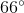II) The base of the tank has a radius of 5 inches.

Either statement is sufficient to answer the question.

Statement II is sufficient to answer the question, but Statement I is not sufficient to answer the question.

Both statements are needed to answer the question.

Statement I is sufficient to answer the question, but Statement II is not sufficient to answer the question.

Statement I is sufficient to answer the question, but Statement II is not sufficient to answer the question.

Explanation:

To find the percentage that the sticker covers, we need to know what portion of the circle it covers. We could find this either with the area of the circle or the area of the sector, but we don't have a good way to find the area of the sector.

Statement I gives us the degree measurement of the sticker. We can make a fraction,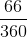, to find the percentage of the base covered.

Statement II gives us the radius of the base, which lets us also know the radius of the sticker; however, this will not be helpful in finding the percentage of the base covered, so Statement II is not really helpful.

Thus, Statement I is sufficient and Statement II is not.

Recap:

Dirk bought a sticker to cover part of the base of his scuba tank. The sticker and the base have the same radius. Given the following, find the percentage of the base that the sticker covers.

I) The central angle of the sticker isII) The base of the tank has a radius of 5 inches

So, according to Statement I, the angle of the sticker is 66 degrees. Because we are dealing with a circle, we can find the percentage of the circle covered by putting 66 over 360.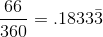The sticker covers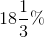of the scuba tank.

### Example Question #2 : Sectors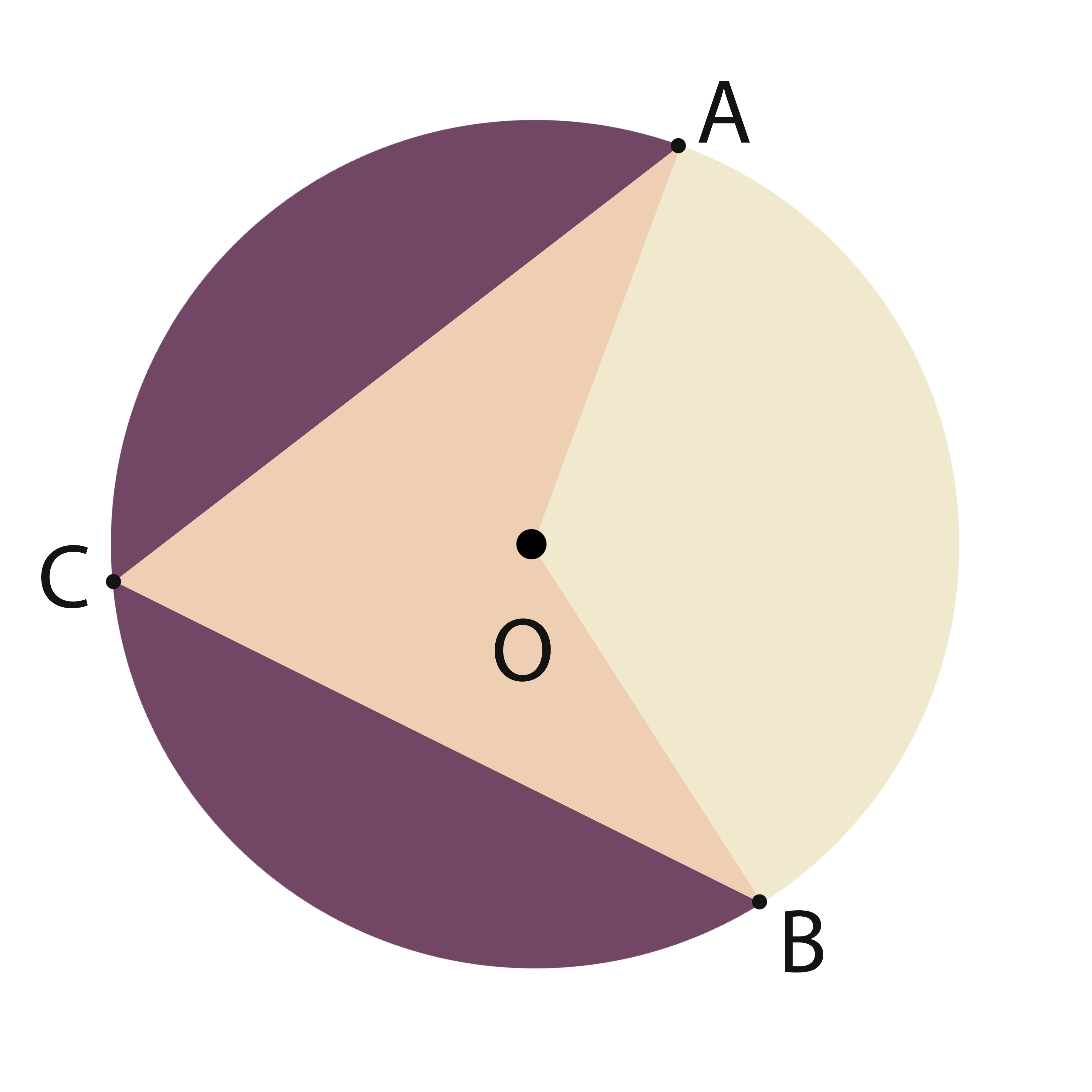What is the area of sector AOB?

1)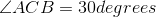2) Arclength of AB is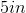Statement 1 alone is sufficient.

Either of the statements is sufficient.

Statement 2 alone is sufficient.

Together the two statements are sufficient.

Neither of the statements, separate or together, is sufficient.

Together the two statements are sufficient.

Explanation:

The angle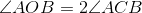, so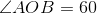.

The Arc length can be related to the radius as follows: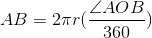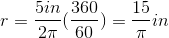From there, the area of the sector can be found: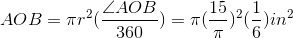### Example Question #3 : Sectors

Consider circle F with sector BFG. What percent of F is BFG?

I) Circle F has a radius of 5 meters and a circumference of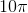meters.

II) Sector BFG has a central angle measure of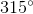.

Either statement is sufficient to answer the question.

Both statements are needed to answer the question.

Statement I is sufficient to answer the question, but statement II is not sufficient to answer the question.

Statement II is sufficient to answer the question, but statement I is not sufficient to answer the question.

Statement II is sufficient to answer the question, but statement I is not sufficient to answer the question.

Explanation:

Consider circle F with sector BFG. What percent of F is BFG?

I) Circle F has a radius of 5 meters and a circumference ofmeters

II) Sector BFG has a central angle measure ofTo find the percentage of a circle covered by a sector, we need to know the central angle. A circle will always have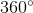, so if we put the angle given to us over. So, II) allows us to answer the question: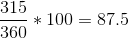So sector BFG is 87.5% of circle F

I) Give us interesting information, but not helpful in regards to the current question. It doesn't matter what the radius is, and the circumference is also irrelevant.

Therefore, II) is sufficient, but I) is not.

### Example Question #4 : Sectors

Jane wants to put a sector-shaped sticker on the bottom of her cylindrical water bottle. The water bottle has a circular base. Find the percent of the base that the sticker covers

I) The sticker has a thickness of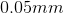.

II) The central angle of the sticker has a measure of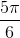.

Either statement is sufficient to answer the question.

Statement I is sufficient to answer the question, but statement II is not sufficient to answer the question.

Statement II is sufficient to answer the question, but statement I is not sufficient to answer the question.

Both statements are needed to answer the question.

Statement II is sufficient to answer the question, but statement I is not sufficient to answer the question.

Explanation:

Jane wants to put a sector-shaped sticker on the bottom of her cylindrical water bottle. The water bottle has a circular base. Find the percent of the base that the sticker covers

I) The sticker has a thickness ofII) The central angle of the sticker has a measure ofI) is irrelevant. The thickness of  the sticker doesn't help us find the area it covers. Statment I) is trying to distract you with 3 dimensions, when we only need to worry about 2.

II) is much more helpful. If we know the central angle, we can find the percentage the sector covers. To find the "answer" perform the following: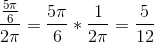So we are dealing with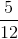of the circle, or 41.67%

Tired of practice problems?

Try live online GMAT prep today.